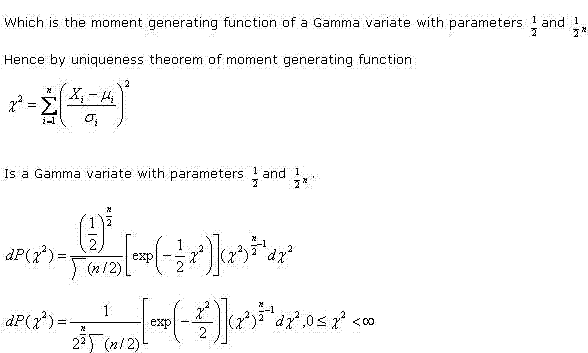# Statistics Assignment Help With Derivation Of The Chi Square Distribution

## 11.2 Derivation Of The Chi Square Distribution:First method – method of moment generating function

If Xi, (i = 1, 2, ……, n) are independent N(μi, σ i2), we want the distribution of

###Which is the required probability density function of chi square distribution with n degrees of freedom.